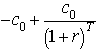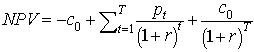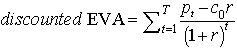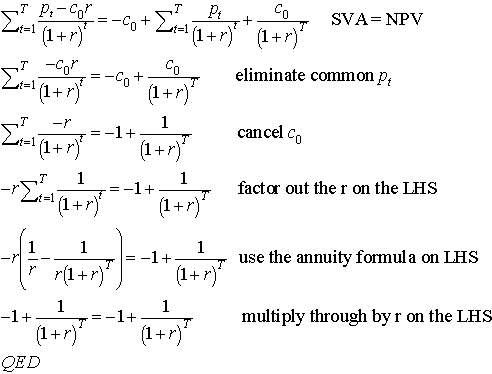EVAÔ is not just a new perfomance measurement fad, it's intimately related to NPV. It's sometmes useful to see the relation. All EVA is simply net after tax income minus a cost of equity capital, and sometimes its better called Net Income After Capital Charge (NIACC). It is a useful way to integrate ROE and net income information, replacing two numbers with one. An important point to remember is that in calculating the discounted EVA, do not count the principal cash flows, i.e., ignore the capital outlays and paypacks. Capital is accounting for by a per period "rent", while NPV does not charge explicitly for the capital other than through the differences between the value of cash today vs. cash tomorrow:.

Discounted EVA is equal to NPV. Not approximates, equals.

Assume an initial capital outlay c0, and payment receipts p every period until time T, discount rate r, and a return of the initial capital outlay. The NPV is thus the following:EVA is simply. The shareholder value added (SVAÔ ) or discounted EVA, isProposition: SVA, or discounted EVA=NPV

proof: